/
/
/
261) f(x, y, z) = x + 2y - 2z,
Not my Question
Flag Content

# Question : 261) f(x, y, z) = x + 2y - 2z, : 1757207

261) f(x, y, z) = x + 2y - 2z, x2 + y2 + z2 = 9

A) Maximum: 1 at (-1, -2, -3); minimum: -1 at (1, 2, 3)

B) Maximum: 9 at (1, 2, -2); minimum: -9 at (-1, -2, 2)

C) Maximum: 8 at (2, 1, -2); minimum: -8 at (-2, -1, 2)

D) Maximum: 1 at (1, -2, -2); minimum: -1 at (-1, 2, 2)

262) f(x, y, z) = x2 + y2 + z2, x + 2y + 3z = 6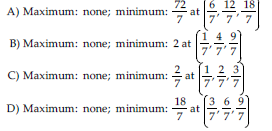263) f(x, y, z) = (x - 1)2 + (y - 2)2 + (z - 2)2, x2 + y2 + z2 = 36

A) Maximum: 77 at (-4, -4, -2); minimum: 13 at (4, 4, 2)

B) Maximum: 49 at (-2, 4, -4); minimum: 41 at (2, -4, 4)

C) Maximum: 81 at (-2, -4, -4); minimum: 9 at (2, 4, 4)

D) Maximum: 77 at (-4, -2, -4); minimum: 13 at (4, 2, 4)

264) f(x, y, z) = x + y + z, 1/x + 1/y + 1/z = 1

A) Maximum: 6 at (2, 2, 2); minimum: -3 at (-1, -1, -1)

B) Maximum: 11 at (6, 3, 2), (6, 2, 3), (3, 2, 6), (3, 6, 2), (2, 3, 6), (2, 6, 3); minimum: 1 at (1, 1, -1), (1, -1, 1), (-1, 1, 1)

C) Maximum: 9 at (3, 3, 3); minimum: 3 at (1, 1, 1)

D) Maximum: 6 at (2, 2, 2); minimum: 3 at (1, 1, 1)

265) f(x, y, z) = 4x - 3y + 2z, x2 + y2 = 6z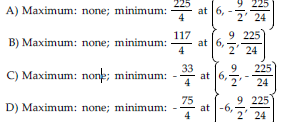266) f(x, y, z) = x2 + 2y2 + 3z2, x - y - z = 1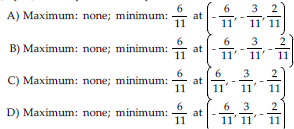267) f(x, y, z) = (x - 2)2 + (y + 1)2 + (z - 4)2, x - y + 3z = 7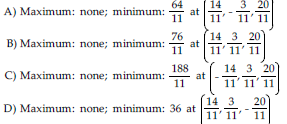268) Find the points on the curve xy2 = 54 that are closest to the origin.

A) (27,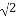) B) (27, ±) C) (3, ±3) D) (3, 3)

269) Find the point on the line x - 3y = 6 that is closest to the origin.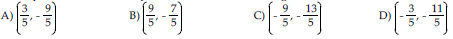270) Find the point on the line 2x + 3y = 5 that is closest to the point (1, 2).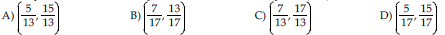## Solution 5 (1 Ratings )

Solved
Mathematics 3 Years Ago 59 Views